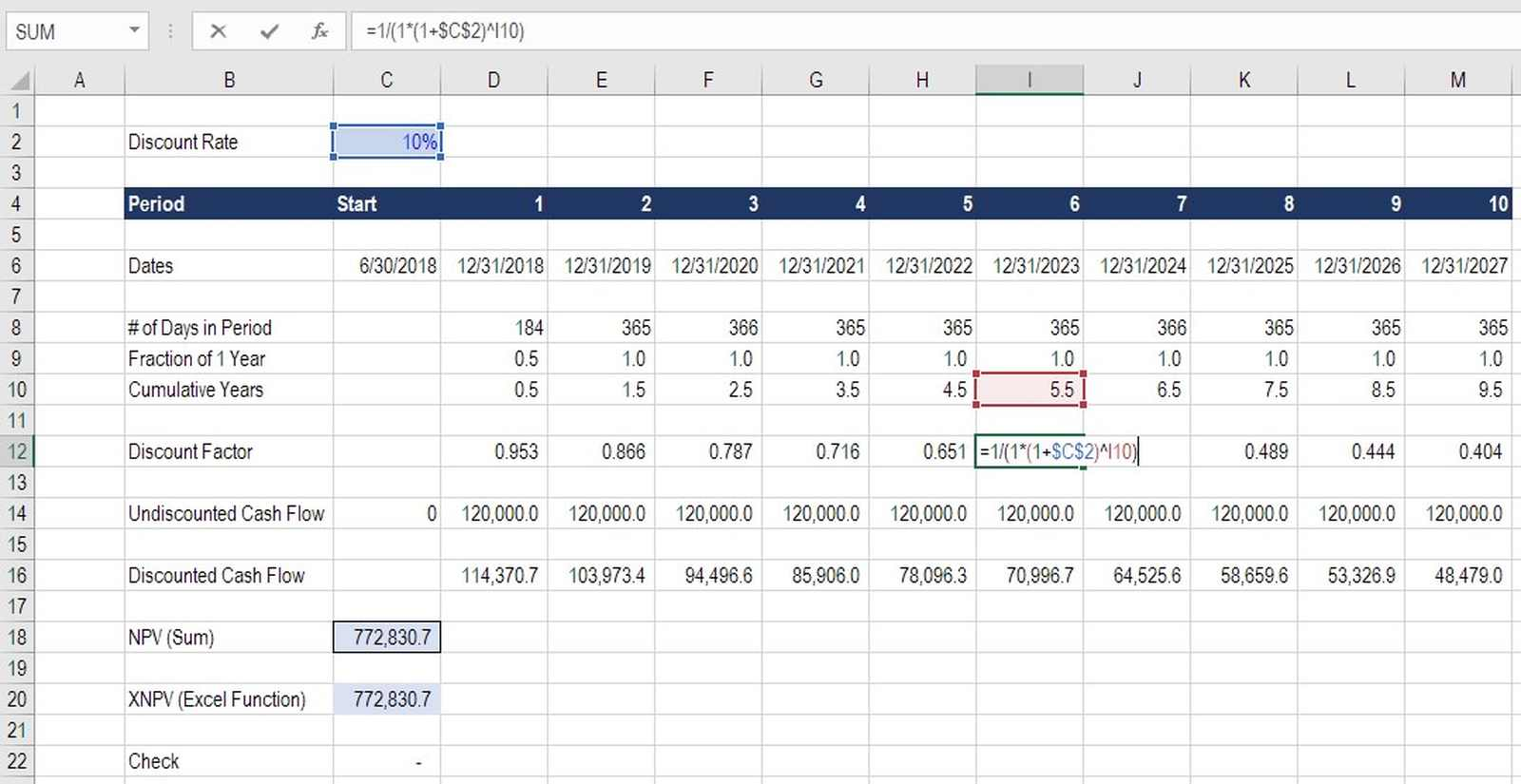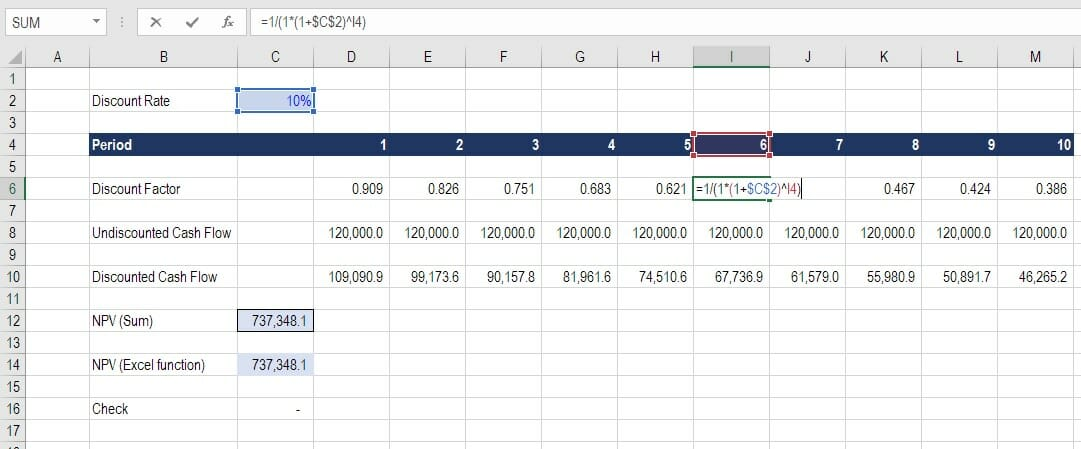# Discount Factor

How to use a discount factor in financial models

## What is a Discount Factor?

In financial modeling, a discount factor is a decimal number multiplied by a cash flow value to discount it back to the present value.  The factor increases over time (meaning the decimal value gets smaller) as the effect of compounding the discount rate builds over time.  Practically speaking, it is easier to use the XNPV function in Excel; however, a benefit of manually calculating the discount factor is that you can see what the present value of each individual cash flow is, as opposed to only the total NPV.### Why Use a Discount Factor?

Some analysts prefer to calculate explicit discount factors in each time period so they can see the effects of compounding more clearly, as well as making the Discounted Cash Flow or DCF model easier to audit.

The discount factor is an alternative to using the XNPV or XIRR functions in Excel.  In the example below, you will see exactly how the discount is used in a spreadsheet.

### Formula for the Discount Factor

The formula for calculating the discount factor in Excel is the same as the Net Present Value (NPV formula).

The formula is as follows:

Factor = 1 / (1 x (1 + Discount Rate) ^ Period Number)

### Sample Calculation

Here is an example of how to calculate the factor from our Excel spreadsheet template.In period 6, which is year number 6 that we are discounting, the number in the formula would be as follows:

Factor = 1 / (1 x (1 + 10%) ^ 6) = 0.564

If the undiscounted cash flow in that period is \$120,000, then to get the present value of that cash flow we multiply it by 0.564 to arrive at \$67,736.9.

The total NPV of the cash flows shown in the example above is \$737,348.1 which can be calculated by summing up the individual discounted cash flows.  We arrive at the same number as using the NPV function in Excel.

### Applications in Financial Modeling

Analysts will use discount factors when performing financial modeling in Excel if they want to have more visibility into the NPV formula and to better illustrate the effect of discounting.

As you see in the above example, every dollar of cash flow received in year 10 is only worth 38.6% of every dollar of cash flow received today.  Once you get more than 15 to 20 years out, the value of cash flows become extremely discounted. As the risk of ever receiving them becomes that much greater, the opportunity cost becomes that much higher.

### Discount Factor vs. XNPV

Using a discount factor allows you to specify exactly how many days are between each period.  You can do this by using specific dates in each time period and taking the difference between them.

For example, June 30, 2018 to December 31, 2018 is 184 days, which is half a year.  By adding this extra layer into the model we can be very precise about our discounting periods.  This achieves the exact same effect as using the XNPV function and XIRR function in Excel.

### More Resources from CFI

In order to keep developing your skills as a world-class financial analyst, we believe these additional resources will be extremely helpful for you:

• Excel formulas and functions
• DCF modeling guide
• XIRR vs IRR
• Free Excel formulas course

### Financial Modeling Certification

Become a certified Financial Modeling and Valuation Analyst (FMVA)® by completing CFI’s online financial modeling classes!